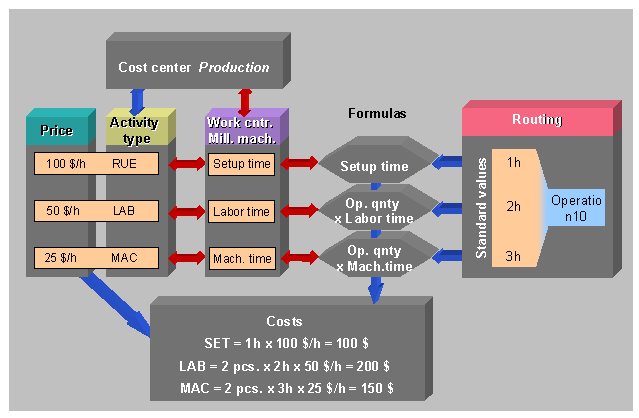Show TOC

###Example: Costing with Cost Centers( ) Costing with Cost Centers

The "Production" cost center has been assigned three activity types. Each activity type was valuated with an activity price. t. In this example, a setup hour (SET) costs \$100.

Since the work center is assigned to a cost center, the activity types (as long as permissible in the cost center) can be assigned to standard values in the work center. In this example the activity types SET, MAC and LAB are assigned to the standard values "Setup", "Machine" and "Labor" in the "Milling machine " work center. These activity types are defaults in all the operations that are executed at this work center.

In the work center a formula can also be assigned to each standard value for costing. Formulas are used to calculate activities for the general operation data (here operation quantity) from the general operation data and the standard values.

The prices for these activity quantities are calculated using the activity prices.